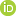Title A drift correction optimization technique for the reduction of the inter-measurement dispersion of isotope ratios measured using a multi-collector plasma mass spectometer Tweet Doherty, W; Lightfoot, P C; Ames, D ESpectrochimica Acta, Part B: Atomic Spectroscopy vol. 98, pt. B, 2014 p. 28-38, https://doi.org/10.1016/j.sab.2014.05.0082014 Earth Sciences Sector, Contribution Series 20140553 Elsevier BV serial English paper; on-line; digital pdf geochemistry; isotopes; solvent extraction; mass spectrometer analysis; spectrographic analysis; isotope ratios graphs; tables; equations Targeted Geoscience Initiative (TGI-4) Mafic-Ultramafic Ore Systems The effects of polynomial interpolation and internal standardization drift corrections on the inter-measurement dispersion (statistical) of isotope ratios measured with a multi-collector plasma mass spectrometer were investigated using the (analyte, internal standard) isotope systems of (Ni, Cu), (Cu, Ni), (Zn, Cu), (Zn, Ga), (Sm, Eu), (Hf, Re) and (Pb, Tl). The performance of five different correction factors was compared using a (statistical) range based merit function ?m which measures the accuracy and inter-measurement range of the instrument calibration. The frequency distribution of optimal correction factors over two hundred data sets uniformly favored three particular correction factors while the remaining two correction factors accounted for a small but still significant contribution to the reduction of the inter-measurement dispersion.Application of the merit function is demonstrated using the detection of Cu and Ni isotopic fractionation in laboratory and geologic-scale chemical reactor systems. Solvent extraction (diphenylthiocarbazone (Cu, Pb) and dimethylglyoxime (Ni)) was used to either isotopically fractionate the metal during extraction using the method of competition or to isolate the Cu and Ni from the sample (sulfides and associated silicates). In the best case, differences in isotopic composition of ± 3 in the fifth significant figure could be routinely and reliably detected for Cu65/63 and Ni61/62.One of the internal standardization drift correction factors uses a least squares estimator to obtain a linear functional relationship between the measured analyte and internal standard isotope ratios. Graphical analysis demonstrates that the points on these graphs are defined by highly non-linear parametric curves and not two linearly correlated quantities which is the usual interpretation of these graphs. The success of this particular internal standardization correction factor was found in some cases to be due to a fortuitous, scale dependent, parametric curve effect. 296192